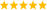# LabVIEW

cancel
Showing results for
Did you mean:

Solved!
Go to solution
Highlighted

## Re: Calculations with long equations

You can further simplify the "pure" LabVIEW  code as shown below. One of the nice things about the Compound Arithmetic node is that you can invert the inputs independently.Message 11 of 17
(247 Views)
Highlighted

## Re: Calculations with long equations

@Yamaeda wrote:

10M loops yields ~700 vs ~100ms (formula node in other case). So no, it's not noticable in a single run. But as i said, if the requirements are such that'll run many times in a tight loop, a factor of 8 is noticable. 🙂

Wow.  I saw closer to a 10x difference.  However, I think it is due to constant folding with the equation you supplied and the Formula Node is not able to do that.  I will have to find time to mess around with it (which might not be hard since I am mostly just waiting for an FPGA to compile).

Message 12 of 17
(241 Views)
Highlighted

## Re: Calculations with long equations

@crossrulz wrote:

@Yamaeda wrote:

10M loops yields ~700 vs ~100ms (formula node in other case). So no, it's not noticable in a single run. But as i said, if the requirements are such that'll run many times in a tight loop, a factor of 8 is noticable. 🙂

Wow.  I saw closer to a 10x difference.  However, I think it is due to constant folding with the equation you supplied and the Formula Node is not able to do that.  I will have to find time to mess around with it (which might not be hard since I am mostly just waiting for an FPGA to compile).

Well, it looks like I was mostly right.  I moved the pi^2 and sqrt(2) out of the Formula Node and the times more mostly comparable.  The "pure" code still has a slight advantage, but not enough that I am going to do statistical analysis on it.

So in summary, using the actual math functions are likely to give you faster results than the Formula Node due to constant folding and parallelism.  Enough to worry?  Well, that depends on your application.

Message 13 of 17
(228 Views)
Highlighted

## Re: Calculations with long equations

Interesting discussion overall. I am still searching for any "long equation", though. 😁

(I've seen equations that had to be wrapped to multiple lines in a publication 🐵LabVIEW Champion. It all comes together inGCentralWhat does "Engineering Redefined" mean??
Message 14 of 17
(193 Views)
Highlighted

## Re: Calculations with long equations

@Alexis_Koul wrote:

I am giving an example of the formula: X,Y,Z and C are my input data from the sensors and 120,12,50 and 30 are constants

A = (120*pi*X)/(12*Y) + (Z*50)/(C+30)

Am I missing something or shouldn't you just cancel the 120 and 12 into 10?  Or are those just example constants and sometimes they don't cancel and you put them in the example anyway?

Message 15 of 17
(158 Views)
Highlighted

## Re: Calculations with long equations

Hello,

The equation that I wrote is a smaller example of what I have in mind 😀

Message 16 of 17
(150 Views)
Highlighted

## Re: Calculations with long equations

I assumed it had something to do with constant folding also, and it rather shows the optimization of LV.

Changing Everything to variables it's less than a factor 2 difference.